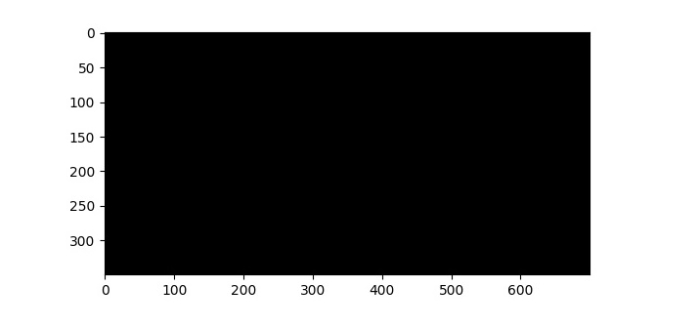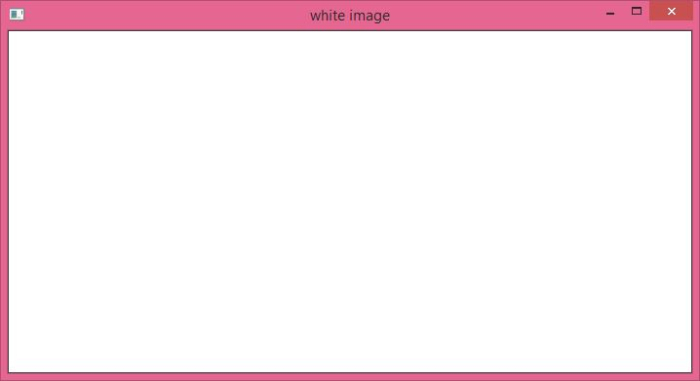# How to create a black image and a white image using OpenCV Python?

To create a black image, we could use the np.zeros() method. It creates a numpy n-dimensional array of given size with all elements as 0. As all elements are zero, when we display it using cv2.imshow() or plt.imshow() functions, it displays a balck image.

To create a white image, we could use the np.ones() method. It creates a numpy n-dimensional array of given size with all elements as 1. We multiply this array by 255 to create a white image. Now all elements are 255, so when we display it using cv2.imshow() or plt.imshow() functions it gives a white image.

Note − While creating numpy.ndarray using np.zeros() or np.ones(), we pass dtype = np.uint8 as an argument.

## Steps

You can follow the steps given below to create a black and a white image −

Import the required libraries. In all the following Python examples, the required Python libraries are OpenCV, NumPy and Matplotlib. Make sure you have already installed them.

import cv2
import matplotlib.pyplot as plt
import numpy as np


Create a numpy.ndarray using np.zeros() to create a black image. Pass the >size and dtype as arguments to these methods. Here dtype is np.uint8.

img = np.zeros((350, 500, 3), dtype = np.uint8)


Create a numpy.ndarray using np.ones() to create a white image. Pass the size and dtype as arguments to these methods. Here dtype is np.uint8. Now we multiply the array by 255.

img = np.ones((350, 500, 3), dtype = np.uint8)
img = 255*img


Display the black or white image.

cv2.imshow('black image', img)


Let's have a look at different examples for clear understanding.

## Example 1

In this example, we create a 700x350 black image. Here the image width is 700 and height is 350.

# import required libraries
import cv2
import numpy as np

# create a black image
img = np.zeros((350, 700, 3), dtype = np.uint8)

# display the image using opencv
cv2.imshow('black image', img)
cv2.waitKey(0)


## Output

When you run the above python program, it will produce the following output window.## Example 2

In this example, we create a 700x350 white image. Note here the image width is 700 and height is 350.

# import required libraries
import cv2
import numpy as np

# create a white image
img = np.ones((350, 700, 3), dtype = np.uint8)
img = 255* img

# display the image using opencv
cv2.imshow('white image', img)
cv2.waitKey(0)


## Output

When you run the above python program, it will produce the following output window.